# 【C++系列】C++实现通讯录管理系统

171 篇文章 18 订阅 ¥9.90 ¥99.00

# 通讯录管理系统

## 1、系统需求

• 添加联系人：向通讯录中添加新人，信息包括（姓名、性别、年龄、联系电话、家庭住址）最多记录1000人
• 显示联系人：显示通讯录中所有联系人信息
• 删除联系人：按照姓名进行删除指定联系人
• 查找联系人：按照姓名查看指定联系人信息
• 修改联系人：按照姓名重新修改指定联系人
• 清空联系人：清空通讯录中所有信息
• 退出通讯录：退出当前使用的通讯录

## 2、创建项目

• 创建新项目
• 添加文件

### 2.1 创建项目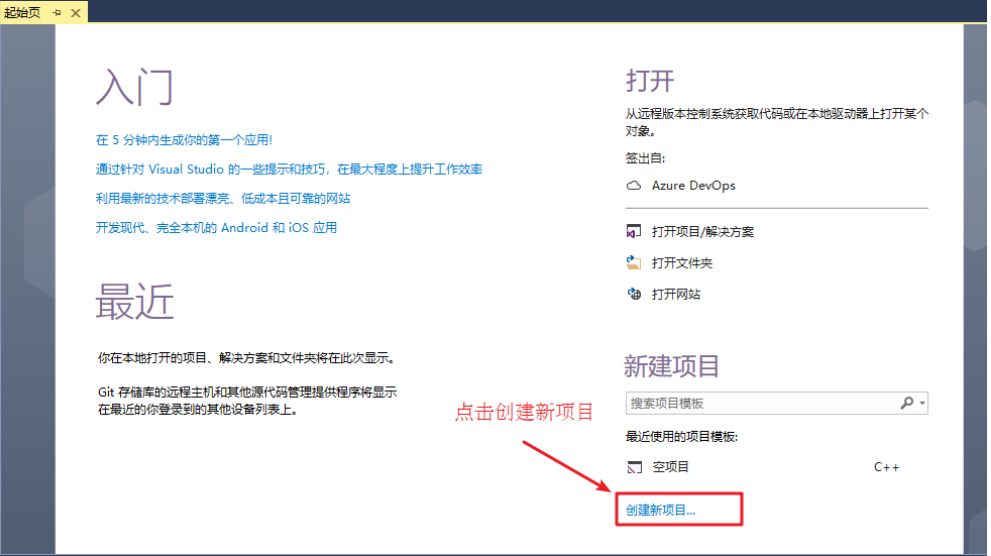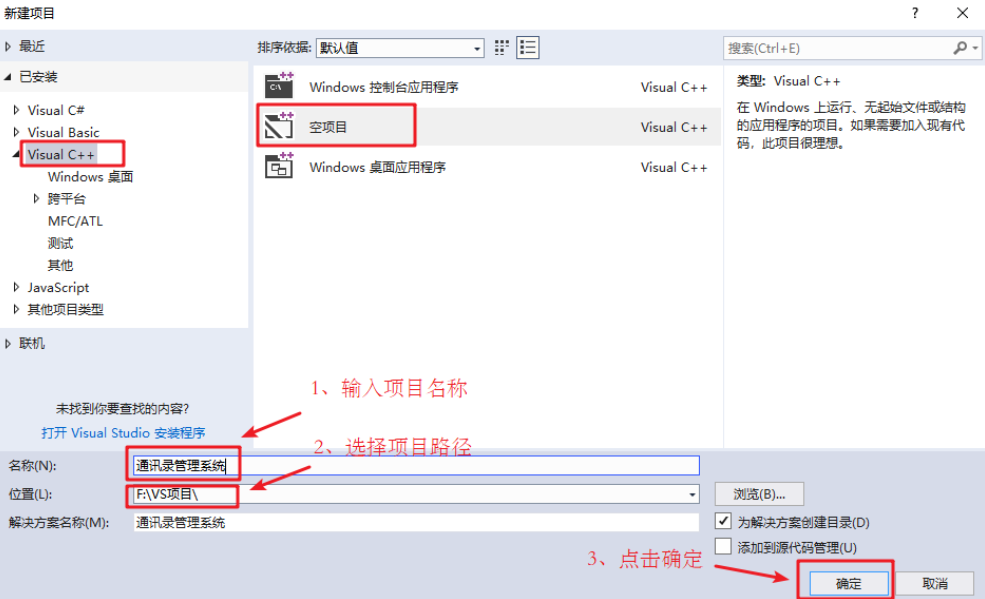### 2.2添加文件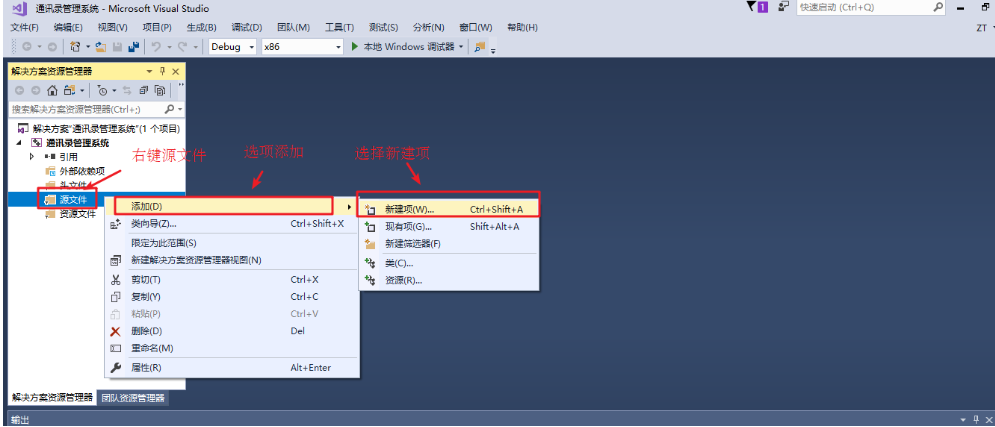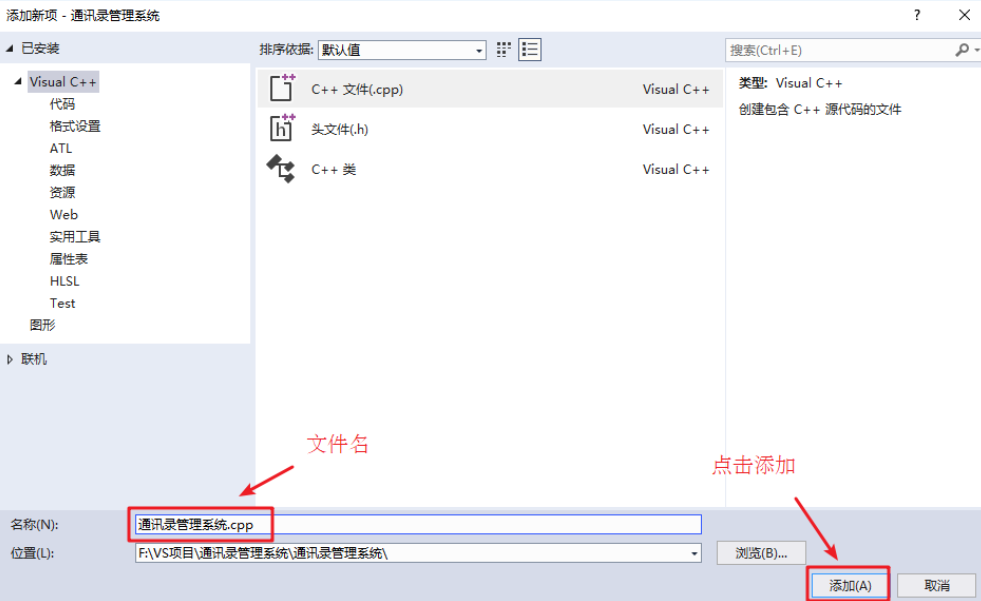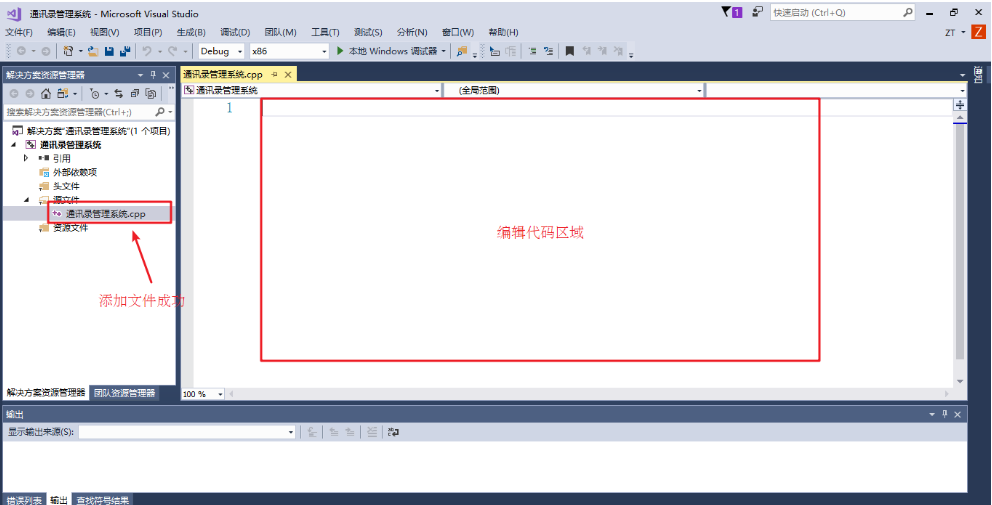## 3、菜单功能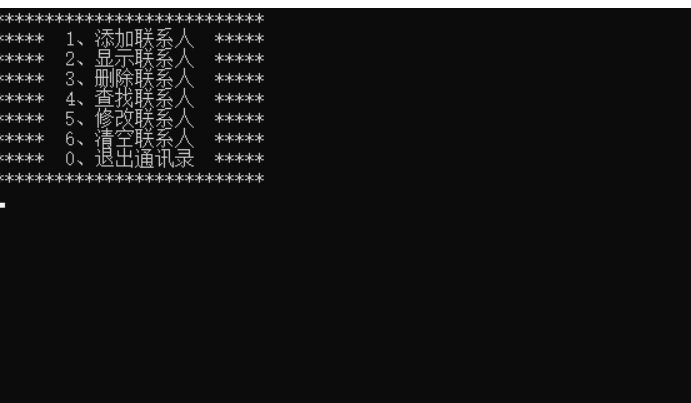• 封装函数显示该界面 如 void showMenu()
• 在main函数中调用封装好的函数

#include<iostream>
using namespace std;

//菜单界面
{
cout << "***************************" << endl;
cout << "*****  1、添加联系人  *****" << endl;
cout << "*****  2、显示联系人  *****" << endl;
cout << "*****  3、删除联系人  *****" << endl;
cout << "*****  4、查找联系人  *****" << endl;
cout << "*****  5、修改联系人  *****" << endl;
cout << "*****  6、清空联系人  *****" << endl;
cout << "*****  0、退出通讯录  *****" << endl;
cout << "***************************" << endl;
}

int main() {

system("pause");

return 0;
}


## 4、退出功能

int main() {

int select = 0;

while (true)
{

cin >> select;

switch (select)
{
case 1:  //添加联系人
break;
case 2:  //显示联系人
break;
case 3:  //删除联系人
break;
case 4:  //查找联系人
break;
case 5:  //修改联系人
break;
case 6:  //清空联系人
break;
case 0:  //退出通讯录
cout << "欢迎下次使用" << endl;
system("pause");
return 0;
break;
default:
break;
}
}

system("pause");

return 0;
}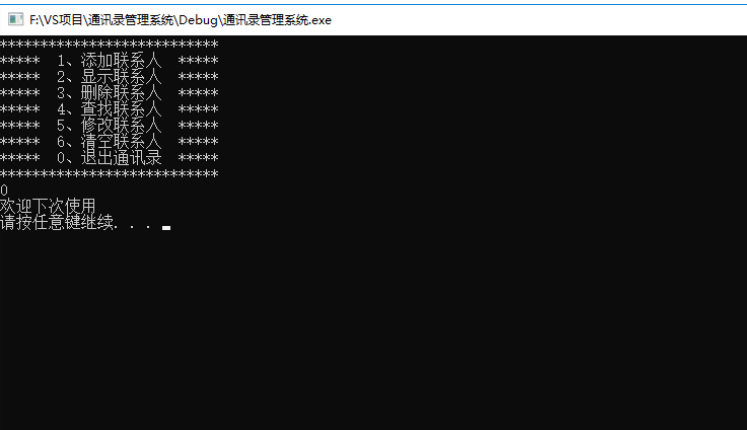## 5、添加联系人

• 设计联系人结构体
• 设计通讯录结构体
• main函数中创建通讯录
• 封装添加联系人函数
• 测试添加联系人功能

### 5.1 设计联系人结构体

#include <string>  //string头文件
//联系人结构体
struct Person
{
string m_Name; //姓名
int m_Sex; //性别：1男 2女
int m_Age; //年龄
string m_Phone; //电话
};


### 5.2 设计通讯录结构体

#define MAX 1000 //最大人数

//通讯录结构体
{
struct Person personArray[MAX]; //通讯录中保存的联系人数组
int m_Size; //通讯录中人员个数
};


### 5.3 main函数中创建通讯录

mian函数起始位置添加：

//创建通讯录
//初始化通讯录中人数
abs.m_Size = 0;


### 5.4 封装添加联系人函数

//1、添加联系人信息
{
//判断电话本是否满了
if (abs->m_Size == MAX)
{
cout << "通讯录已满，无法添加" << endl;
return;
}
else
{
//姓名
string name;
cout << "请输入姓名：" << endl;
cin >> name;
abs->personArray[abs->m_Size].m_Name = name;

cout << "请输入性别：" << endl;
cout << "1 -- 男" << endl;
cout << "2 -- 女" << endl;

//性别
int sex = 0;
while (true)
{
cin >> sex;
if (sex == 1 || sex == 2)
{
abs->personArray[abs->m_Size].m_Sex = sex;
break;
}
cout << "输入有误，请重新输入";
}

//年龄
cout << "请输入年龄：" << endl;
int age = 0;
cin >> age;
abs->personArray[abs->m_Size].m_Age = age;

//联系电话
cout << "请输入联系电话：" << endl;
string phone = "";
cin >> phone;
abs->personArray[abs->m_Size].m_Phone = phone;

//家庭住址
cout << "请输入家庭住址：" << endl;

//更新通讯录人数
abs->m_Size++;

cout << "添加成功" << endl;
system("pause");
system("cls");
}
}


### 5.5 测试添加联系人功能

case 1:  //添加联系人
break;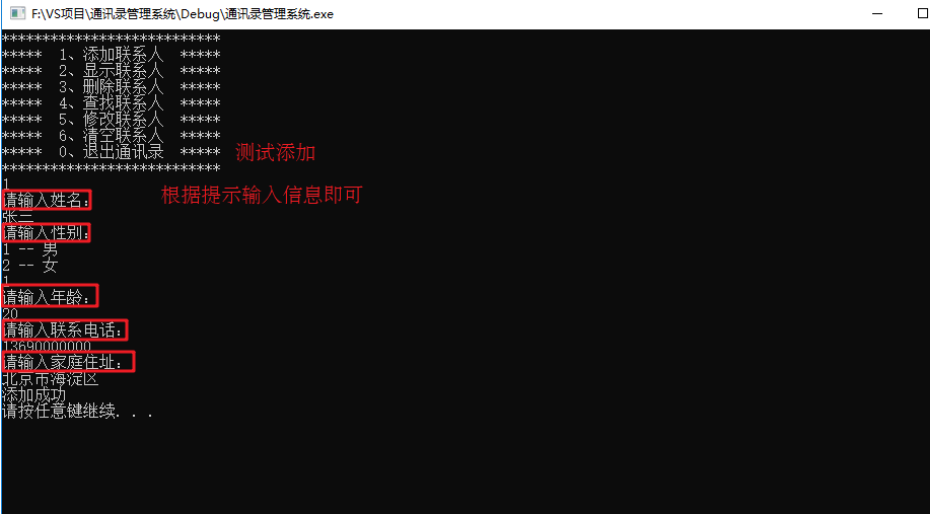## 6、显示联系人

• 封装显示联系人函数
• 测试显示联系人功能

### 6.1 封装显示联系人函数

//2、显示所有联系人信息
{
if (abs->m_Size == 0)
{
cout << "当前记录为空" << endl;
}
else
{
for (int i = 0; i < abs->m_Size; i++)
{
cout << "姓名：" << abs->personArray[i].m_Name << "\t";
cout << "性别：" << (abs->personArray[i].m_Sex == 1 ? "男" : "女") << "\t";
cout << "年龄：" << abs->personArray[i].m_Age << "\t";
cout << "电话：" << abs->personArray[i].m_Phone << "\t";
cout << "住址：" << abs->personArray[i].m_Addr << endl;
}
}

system("pause");
system("cls");

}


### 6.2 测试显示联系人功能

case 2:  //显示联系人
showPerson(&abs);
break;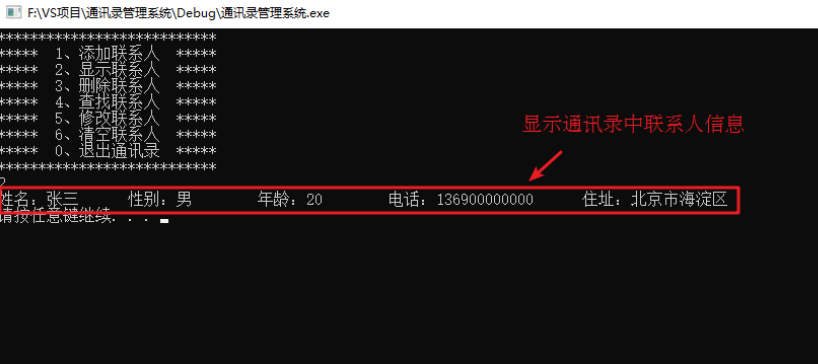## 7、删除联系人

• 封装检测联系人是否存在
• 封装删除联系人函数
• 测试删除联系人功能

### 7.1 封装检测联系人是否存在

//判断是否存在查询的人员，存在返回在数组中索引位置，不存在返回-1
int isExist(Addressbooks * abs, string name)
{
for (int i = 0; i < abs->m_Size; i++)
{
if (abs->personArray[i].m_Name == name)
{
return i;
}
}
return -1;
}



### 7.2 封装删除联系人函数

//3、删除指定联系人信息
{
cout << "请输入您要删除的联系人" << endl;
string name;
cin >> name;

int ret = isExist(abs, name);
if (ret != -1)
{
for (int i = ret; i < abs->m_Size; i++)
{
abs->personArray[i] = abs->personArray[i + 1];
}
abs->m_Size--;
cout << "删除成功" << endl;
}
else
{
cout << "查无此人" << endl;
}

system("pause");
system("cls");
}



### 7.3 测试删除联系人功能

case 3:  //删除联系人
deletePerson(&abs);
break;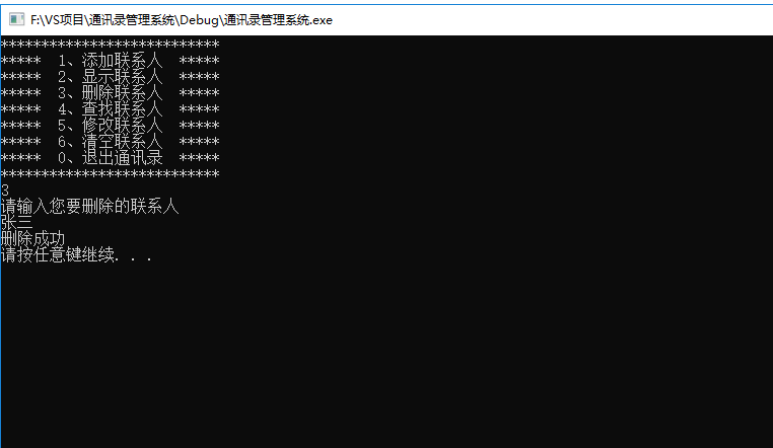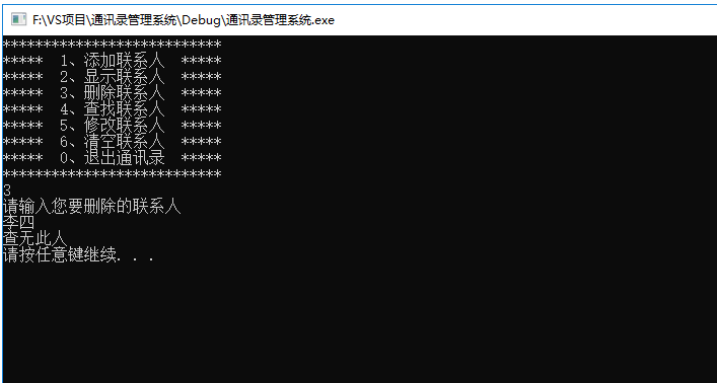## 8、查找联系人

• 封装查找联系人函数
• 测试查找指定联系人

### 8.1 封装查找联系人函数

//4、查找指定联系人信息
{
cout << "请输入您要查找的联系人" << endl;
string name;
cin >> name;

int ret = isExist(abs, name);
if (ret != -1)
{
cout << "姓名：" << abs->personArray[ret].m_Name << "\t";
cout << "性别：" << abs->personArray[ret].m_Sex << "\t";
cout << "年龄：" << abs->personArray[ret].m_Age << "\t";
cout << "电话：" << abs->personArray[ret].m_Phone << "\t";
cout << "住址：" << abs->personArray[ret].m_Addr << endl;
}
else
{
cout << "查无此人" << endl;
}

system("pause");
system("cls");

}


### 8.2 测试查找指定联系人

case 4:  //查找联系人
findPerson(&abs);
break;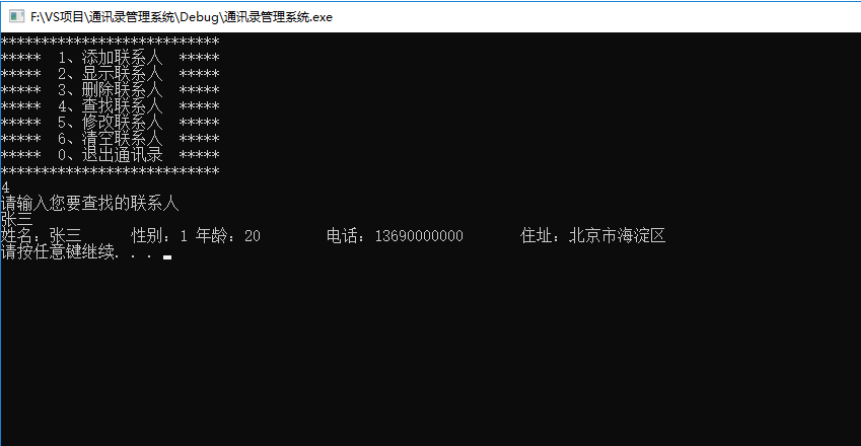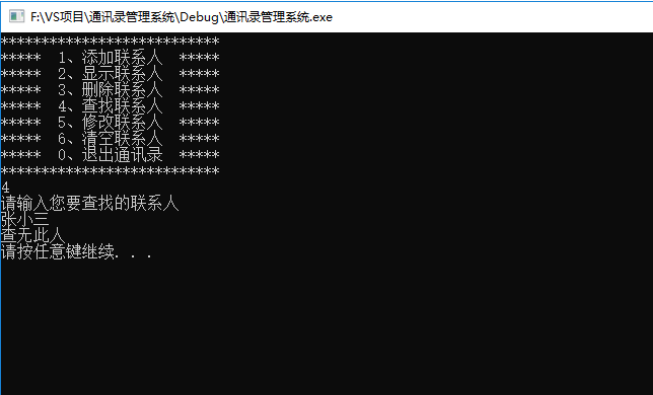## 9、修改联系人

• 封装修改联系人函数
• 测试修改联系人功能

### 9.1 封装修改联系人函数

//5、修改指定联系人信息
{
cout << "请输入您要修改的联系人" << endl;
string name;
cin >> name;

int ret = isExist(abs, name);
if (ret != -1)
{
//姓名
string name;
cout << "请输入姓名：" << endl;
cin >> name;
abs->personArray[ret].m_Name = name;

cout << "请输入性别：" << endl;
cout << "1 -- 男" << endl;
cout << "2 -- 女" << endl;

//性别
int sex = 0;
while (true)
{
cin >> sex;
if (sex == 1 || sex == 2)
{
abs->personArray[ret].m_Sex = sex;
break;
}
cout << "输入有误，请重新输入";
}

//年龄
cout << "请输入年龄：" << endl;
int age = 0;
cin >> age;
abs->personArray[ret].m_Age = age;

//联系电话
cout << "请输入联系电话：" << endl;
string phone = "";
cin >> phone;
abs->personArray[ret].m_Phone = phone;

//家庭住址
cout << "请输入家庭住址：" << endl;

cout << "修改成功" << endl;
}
else
{
cout << "查无此人" << endl;
}

system("pause");
system("cls");

}


### 9.2 测试修改联系人功能

case 5:  //修改联系人
modifyPerson(&abs);
break;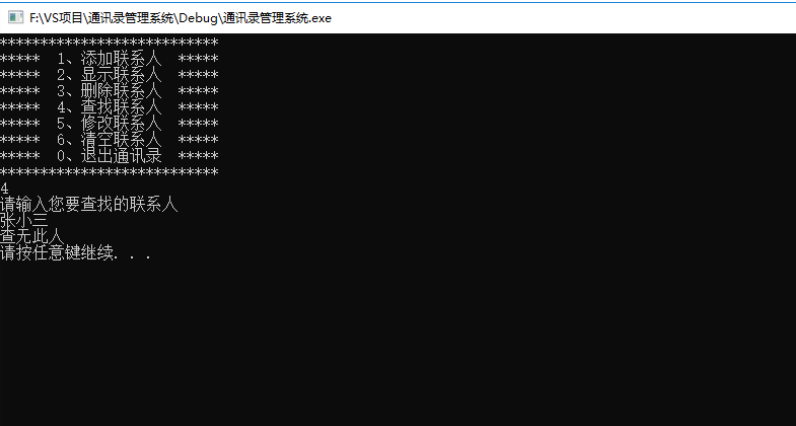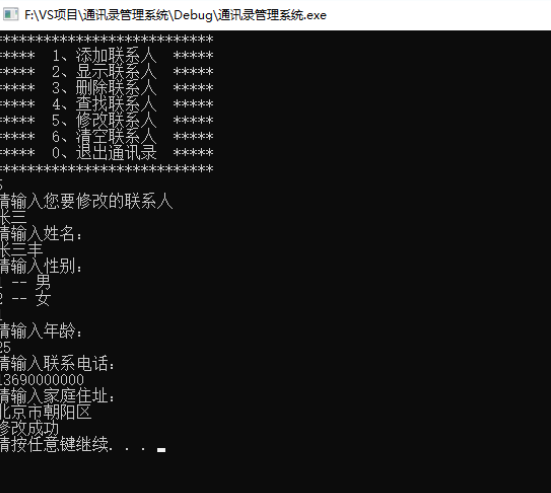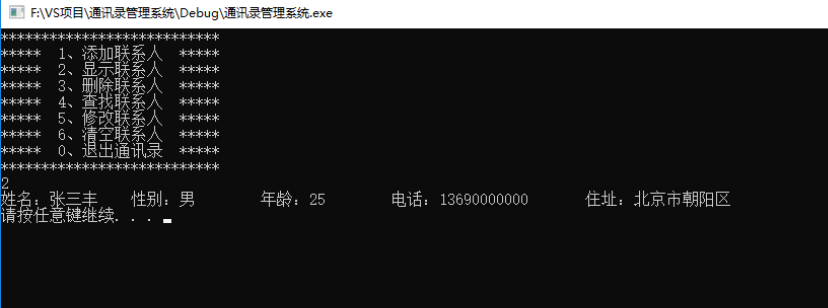## 10、清空联系人

• 封装清空联系人函数
• 测试清空联系人

### 10.1 封装清空联系人函数

//6、清空所有联系人
{
abs->m_Size = 0;
cout << "通讯录已清空" << endl;
system("pause");
system("cls");
}


### 10.2 测试清空联系人

case 6:  //清空联系人
cleanPerson(&abs);
break;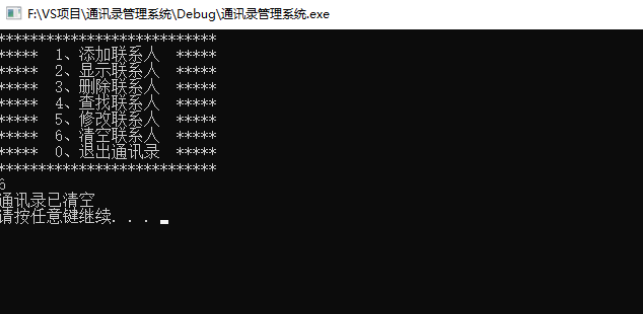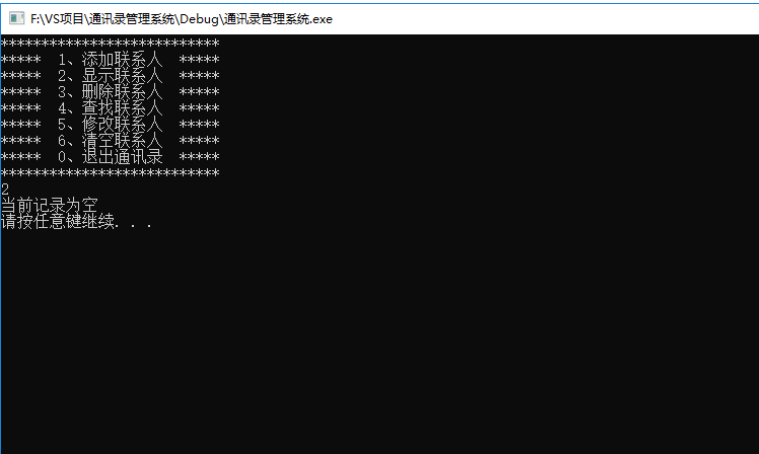01-04
01-08113
11-23454
01-0367
10-111063
06-267079
11-22761
03-201889
05-18216
04-162246
06-24885
11-29922
07-30392
12-091151
06-172385

### “相关推荐”对你有帮助么？

•非常没帮助
•没帮助
•一般
•有帮助
•非常有帮助被折叠的  条评论 为什么被折叠?到【灌水乐园】发言点击重新获取扫码支付1.余额是钱包充值的虚拟货币，按照1:1的比例进行支付金额的抵扣。
2.余额无法直接购买下载，可以购买VIP、C币套餐、付费专栏及课程。余额充值